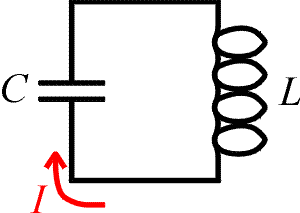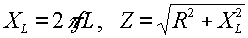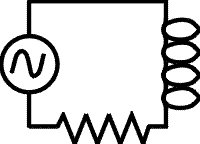Examples for AC circuits

Example #1Problem:

Consider the LC circuit to the right. If one needs to tune this circuit to a frequency of 84 kHz, and the capacitor has a capacitance C = 3.0 mF, what inductance L is needed?

Solution:

Use the relation,. Solve for L.

L = 1.20E-6 H

Example #2Problem:

a.) What is the impedance of the circuit to the right if: f = 60 Hz, L = 20 mH, R = 4.0 W?

Solution:Z = 8.54 W

b.) If the r.m.s. voltage of the source is Vrms = 110 V, what is the r.m.s. current?

Solution: Irms = Vrms/ Z

Irms = 12.9 amps

c.) What is the peak current?

Solution: Ip = sqrt(2) ·Irms

Ip = 18.2 amps

d.) What is the power dissipated in the resistor?

Solution: P = I2rms·R

P = 1320 W

AC circuit's index